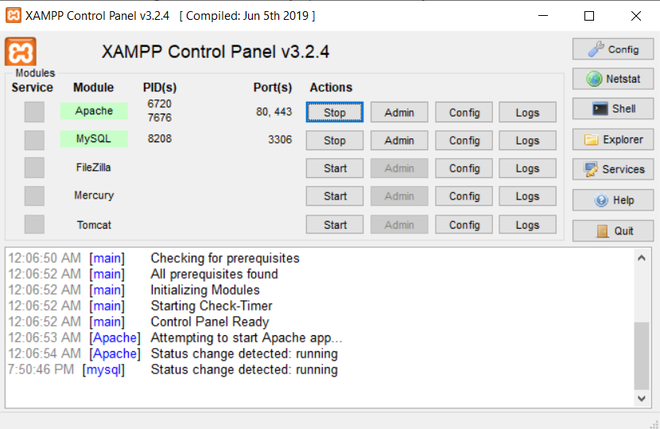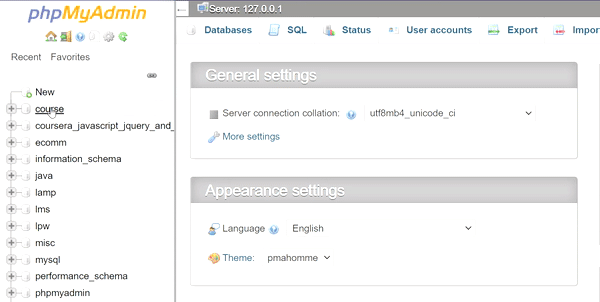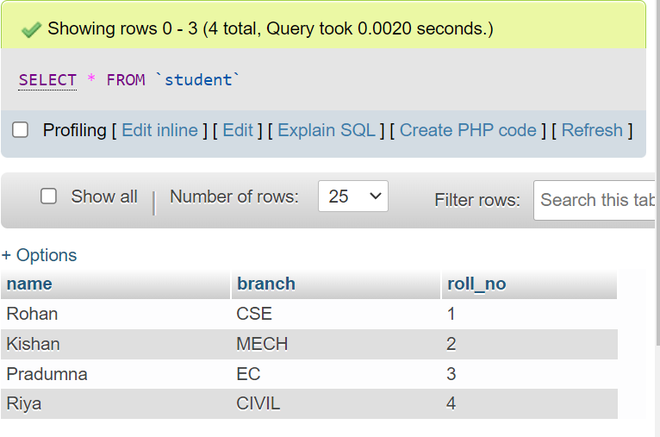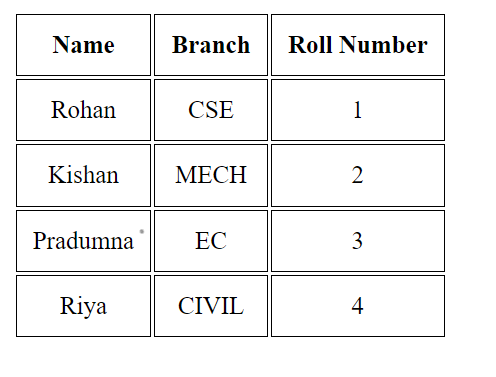# How to retrieve data from MySQL database using PHP ?

• Difficulty Level : Easy
• Last Updated : 10 Mar, 2022

There are steps to understand for retrieving the data from the MySQL database

Approach:

Now we understand each and every step as shown below.

Example 1: In this. we use PHPMyAdmin for the database handling. Start the server in the XAMPP as shown in the below image

Making sure that both Apache and MySQL are showing green color, means that the server is working.XAMPP PANEL

After that create the database in the PHPMyAdmin. Open the below URL.

`http://localhost/phpmyadmin/index.php`

Creating the database:creating the database in MySQL PHPMyAdmin

Create the table: Execute the SQL query in the “gfg” database.

```CREATE TABLE student
(
name varchar(20),
branch varchar(20),
roll_no INT
);```
```INSERT INTO `student` ( `name`, `branch`, `roll_no`)
VALUES ( 'Rohan', 'CSE', '1' );```

After the data is inserted, the table will look like this.After entering the data in the table

PHP Code: Run the PHP script as shown below to fetch the data from the database.

## PHP

 ` connect_errno) ` `    ``{ ` `       ``echo` `"Failed to connect to MySQL: "` `. ``\$conn` `-> connect_error; ` `       ``exit``(); ` `    ``} ` ` `  `    ``\$sql` `= ``"select * from student"``; ` `    ``\$result` `= (``\$conn``->query(``\$sql``)); ` `    ``//declare array to store the data of database ` `    ``\$row` `= [];  ` ` `  `    ``if` `(``\$result``->num_rows > 0)  ` `    ``{ ` `        ``// fetch all data from db into array  ` `        ``\$row` `= ``\$result``->fetch_all(MYSQLI_ASSOC);   ` `    ``}    ` `?> ` ` `  ` ` ` ` ` ` ` `  ` ` `    `` ` `        ``
NameBranchRoll Number
` `            `` ` `                `` ` `                `` ` `                `` ` `            `` ` `        `` ` `        ``
` `            `` ` `            `` ` ` `  `                `` ` `                `` ` `                `` ` ` `  `            `` ` `            `` ` `        `` ` `    `` ` ` ` ` ` ` `  ``

Output:Output of the above PHP script

My Personal Notes arrow_drop_up
Recommended Articles
Page :